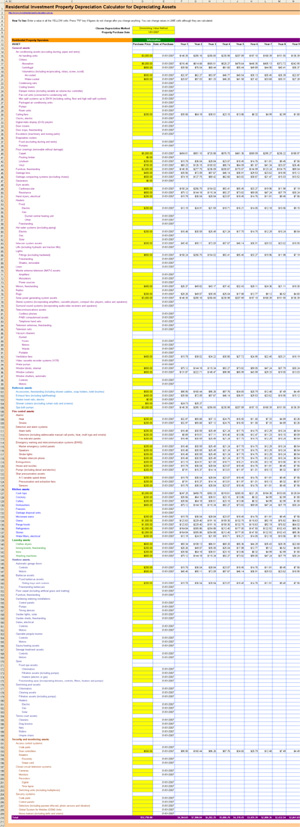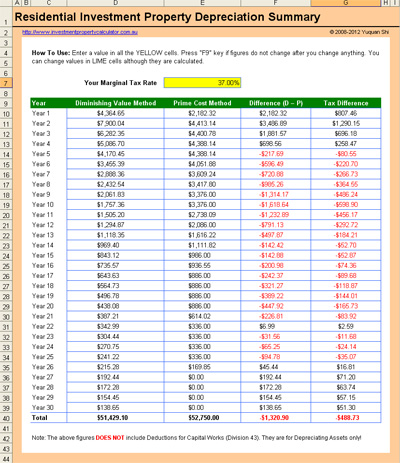Affiliate Program

ULTIMATE
Investment Property Calculator

[ v1.0.2019 ] - [ cost: \$149.99 ] - [ details ]

PROFESSIONAL
Investment Property Calculator

[ v1.0.2019 ] - [ cost: \$64.99 ] - [ details ]

STANDARD
Investment Property Calculator

[ v1.0.2019 ] - [ cost: \$44.99 ] - [ details ]

FREE
Investment Property Calculator

[ v1.0.2019 ] - [ cost: \$0.00 ] - [ details ]

\$190 PROPERTY
Depreciation Schedule Report

[ hot deal ] - [ cost: from \$190.00 ] - [ details ]

COMMERCIAL PROPERTY

[ v1.0.2019 ] - [ cost: \$299.99 ] - [ details ]

FREE Investment Property Depreciation Calculator

This Investment Property Depreciation Calculator is developed to help Australia property investors in estimating the tax depreciation deductions of the depreciating assets so that they can make more informed decisions on their residential rental property invesetment activities.

This investment property depreciation calculator is built based on ATO's Guide to Depreciating Assets. Here is a list of features that this free tax depreciation calculator provides.

1. Allows to specify purchase price for each depreciating asset;

2. Allows to swith betwee the diminishing value method and the prime cost method;

3. Estimates 30 years annual tax depreciation for depreciating assets (the annual decline in value);

4. Generates a summary table which show the difference betwee the diminishing value method and the prime cost method in terms of depreciation value and tax;

5. Built-in first year apportionment based on the property purchase date;

6. Suits all kinds of properties including renovated or fully furnished;

This calculator is built in Microsoft Excel worksheet. You need to have Microsoft Excel 2000-2010 and Microsoft Windows to use it. You may be able to use the calculator with other applications that can open and read XLS spreadsheets, but this has not been tested.

Here is a screen shot that will give you a better idea that what you need to do and how this Investment Property Depreciation Calculator can help you.View full size image of Investment Property Depreciation CalculatorView full size image of Investment Property Depreciation Calculator - Summary

You don't have to purcahse a depreciation schedule before you make any desision by using this free depreciation calculator to estiamte property depreciation for your potential rental property although you have to use the investment property depreciation from a formal depreciation schedule from a qualified quantity surveryor when doing your tax return.

There are two methods on how to calculate rental property depreciation: diminishing value method and prime cost method.

Here are the ATO definitions of the diminishing value method and prime cost method:

The diminishing value method assumes the decline in value each year is a constant proportion of the remaining value and produces a progressively smaller decline over time.

The diminishing value method decline in value deductible amount of a depreciating asset for an income year is the opening adjustable value divided by either:

(a) the effective life, multiplied by 1.5. This applies to the assets you started to hold before 10 May 2006, or

(b) the effective life, multiplied by 2.0. This applies to the assets you started to hold on or after the 10 May 2006,

reduced by your percentage of non-taxable use of the asset.

The prime cost method assumes that the value of a depreciating asset decreases uniformly over its effective life.

The prime cost method decline in value of a depreciating asset for an income year deductible amount is the lesser of:

(a) the cost divided by the effective life apportioned for any part year ownership, and